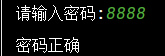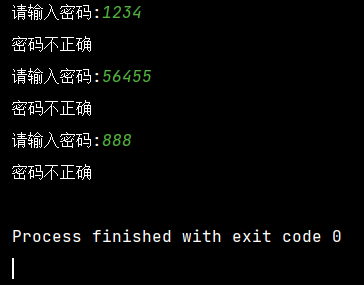### python学习总结part3

• 前言
• 一、笔记
• 1.变量的作用域
• 2.len-获得字符串的长度
• 3.pow函数
• 4.round函数
• 5.while-if嵌套循环
• 6.for-if嵌套循环
• 总结

# 一、笔记

## 1.变量的作用域

def goldsec():

globao x    #声明x为全局变量
x=9000

x=1
print(x)
goldsec()
print(x)

## 2.len-获得字符串的长度

length=0

while length==0:

length=len(name)

print('hello',name)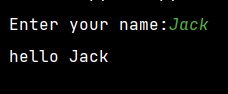## 3.pow函数

pow(a,b)-----求a的b次方

a=int(input('Enter a number:'))
b=int(input('And another'))

print(a,'to the power of',b,'is',pow(a,b))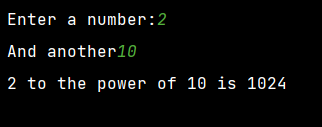## 4.round函数

round(a,b)-----将a精确到小数点后b位

a=float(input('Enter a number:'))

print(round(a,3))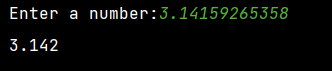## 5.while-if嵌套循环

round(a,b)-----将a精确到小数点后b位

a=0
while a<3:
a += 1
pwd=input('请输入密码:')
if pwd=='8888':
print('密码正确')
break
else:
print('密码不正确')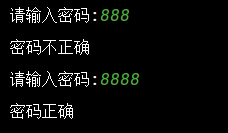## 6.for-if嵌套循环

for item in range(3):
pwd=input('请输入密码:')
if pwd=='8888':
print('密码正确')
break
else:
print('密码不正确')